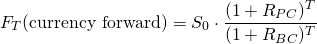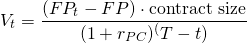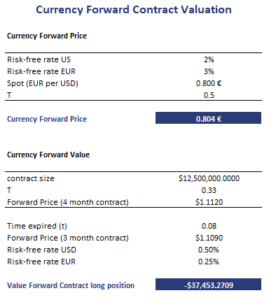# Currency Forward Valuation

The pricing and valuation of currency forward contracts uses the covered interest rate parity to determine the no-arbitrage price. In particular, higher interest rate currencies will trade at a forward discount to interest rates with lower interest rates. The forward discount will offset the extra interest income earned by the higher interest rate currencies.

On this page, we discuss how to calculate the forward price of a currency forward and also how to value the contract after initiation. We also implement an example that uses an Excel spreadsheet. The Excel file can be downloaded at the bottom of the page.

## Currency forward pricing formula

Before we discuss the valuation of currency forward contracts, let’s first discuss how to price them.The formula to price a currency forward contract is the followingwhere F and S are the forward and spot price in price currency per unit of base currency. Rpc is the price currency interest rate, while Rbc is the base currency interest rate. The above formula gives us the no-arbitrage forward price of one unit of foreign currency, in terms of the home currency, for a currency forward that expires in T years. Keep in mind that currency forward contracts use a 365-day convention.

## Currency forward valuation formula

Next, there’s the value of the contract after initiation. To value the contract, we need to use the following formulawhere FP is the forward price at initiation, FPt is the forward price of a contract at time t for a contract maturing at T, and rpc is the interest rate of the price currency.

## Currency forward valuation example

Finally, let’s turn to an example that illustrates how to use the above formulas. The table below implements the two formulas presented above. That is, we calculate both the price of a currency forward contract as well as the value of a currency forward contract using two seperate examples.The Excel file is available for download at the bottom of the page.

## Summary

We discussed the pricing and valuation of currency forward contracts.